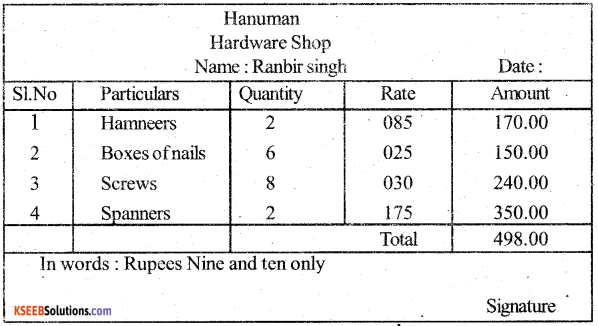# KSEEB Solutions for Class 5 Maths Chapter 5 Money

Students can Download Maths Chapter 5 Money Questions and Answers, Summary, Notes Pdf, KSEEB Solutions for Class 5 Maths helps you to revise the complete Karnataka State Board Syllabus and score more marks in your examinations.

## Karnataka State Syllabus Class 5 Maths Chapter 5 Money

I. Find the following:

1. Sum of Rs. 13.25, Rs.6.30, Rs 10.40 = ____________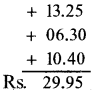2. Difference of Rs,78.45 and Rs.69.70 = ___________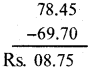3. Product of Rs.147.25 and Rs 17 = _____________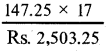4. Quotient of Rs. 4960 ÷ Rs. 8 = 620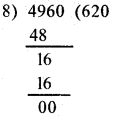II. Solve the following:

Question 1.
Subtract Rs. 95.80 from the sum of Rs.279.05 and Rs.103.25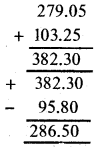Question 2.
Express Rs.32 in paise.
1Rs= 100 Paise
32 Rs = $$\frac{100 \times 32}{3200 \mathrm{Paise}}$$

Question 3.
Express Rs. 19.75 in paisa
1 Rs = 100 Paise
19.75 Rs = $$\frac{19.75 \times 100}{1,975.00 \text { Paise }}$$Question 4.
Express 4,705 paise in rupees.
100 Paise = 1 Rupee.
4,705 Paise = $$\frac{4705}{100}$$
= Rs. 47.05

### KSEEB Class 5 Maths Money Ex 5.1

Problems involving addition in Money system.

I. Solve:

Question 1.
I had Rs. 625 with me. My father gave Rs. 450.75 as pocket money. What is the total amount with me now?
My father gave – Rs 450.75
The total Amounts with me – Rs 1,075.75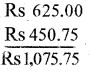Question 2.
Mahesh buys a pen for Rs. 18.5, a book for Rs.10.75 and a bag for Rs.125. What is the total cost of all the things bought?
Mahesh buys a pen – Rs 18.50
Mahesh buys a book – Rs 10.75
Mahesh buys a Bag – Rs 125.00
The total cost of all thing – Rs. 154.25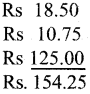Question 3.
Razia had Rs. 345 with her. She bought a dress for Rs. 245.75. What is the amount left with her?
Girija buys 2 Kg of beans – Rs 36.50
Girijabuys 1 Kg of tomatoes – Rs 12.25
Girija buys 1 Kg of potatoes – Rs 14.75 6
The total Amount Girija pay to the shopkeeper – Rs 63.50

Problems involving subtraction in Money system.

II. Solve:

Question 1.
Subtract Rs. 348.25 from Rs. 500.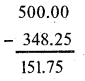Question 2.
Varun bought sweets for Rs.475.5. He paid Rs.500 to the shop keeper. What change should he get back from the shopkeeper?
Varun paid – Rs 500.00
Varun bought sweets – Rs 475.50
Varun get back from the shopkeeper – Rs 124.50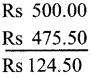Question 3.
Razia had Rs.345 with her. She bought a dress for Rs.245.75. What is the amount left with her?
Razia bought a dresses – Rs 245.75
Razia amount left – Rs 99.25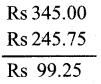Question 4.
Poornima had Rs.3,425 with her. She gave Rs.2,745.75 to her friend to buy a saree. What is the amount left with her?
Poornima had an amount – Rs 3,425.00
Poornima gave an amount – Rs 2,745.75
To her friend
The amount left with Poornima Rs 679.25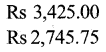Question 5.
Joseph had Rs. 2,500 in the account in a bank. He withdrew Rs. 1,800.75 for his expenses. What is the amount left in his account in the bank?
Joseph had amount in a bank – Rs 2500.00
Joseph withdraw amount in a bank – Rs 1800.75
The amount left with joseph – Rs 699.25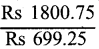Problems involving Multiplication in Money system.

III. Solve:

Question 1.
The cost of an egg is Rs.4. What is the cost of a dozen eggs?
The cost of an egg – Rs 4
The cost of a dozen egg – Rs 12 × 4
The amount of a dozen eggs – Rs 48Question 2.
Swathi buys 8 metres of cloth at Rs.75 per metre. What is the amount to be paid to the shopkeeper?
Swathi buy a cloth Rs 75 per metre
Swathi buys 8 metre of cloth – 75 x 8
Swathi paid to the shopkeeper – Rs 600

Question 3.
The cost of an umbrella is Rs.225 What is the cost of 15 umbrellas?
The cost of an umbrella – Rs 225
The cost of 15 umbrella as 225 × 15 = 3,375

Question 4.
A labourer earns Rs.525 a day. How much will he earn in a week?
A labourer earns amount – Rs 525
A labourer earns amount –  Rs 525 × 7
In a week = 3675

Question 5.
The cost of a table is Rs.4,320. What is the cost of 16 tables?
The cost of a table Rs 4,320
The cost of a table Rs 4,320 × 16 = 69,120

Problems involving division in Money System

IV. Solve:

Question 1.
The Government higher primary school sanctioned a scholarship amount of Rs.4,000 for 10 students, How much amount did each one get?
The government higher primary school sanctioned a scholarship amount – Rs 4,000
For 10 students – Rs 4,000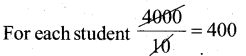For each students gets – Rs 400

Question 2.
Mohan paid Rs. 1,422 for 9 shirts. What is the cost of each shirt?
Mohan paid Rs 1,422 for 9 shirts
The cost of each shirts $$\frac{1422}{9}$$
The cost of Shirts Rs 158

Question 3.
Distribute Rs.4,425 among Amar, Akbar and Anthony equally. What is the amount of share that each one gets?
Distribute Rs 4,425 among Akhbar Amar & Anthony equally
The amount of share each gets = $$\frac{4425}{3}$$ = 1475 RsQuestion 4.
If the cost of a dozen apples is Rs. 96, what is the cost of one apple?
The cost of a dozen apples = 96
The cost of one apple $$\frac{96}{12}$$ = 8 Rs

Question 5.
Vinod, a carpenter earns Rs.9,666 in 6 days. What is the amount he earns in a day?
Vinod a carpenter earns 9.666 in 6 days
The amount vinod earns a day = $$\frac{9666}{6}$$ = 1611 Rs

### KSEEB Class 5 Maths Money Ex 5.3

Miscellaneous Problems in Money System

I. Solve:

Question 1.
Subtract Rs. 687.25 from the sum of Rs.932.60 and Rs.403.7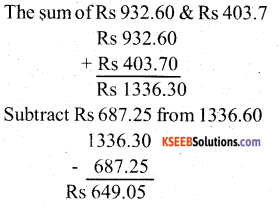Question 2.
Ramegowda had Rs.8,000 with him. He spent Rs.2,450.25 to buy mango saplings, Rs.675.5 for seedlings and Rs.150 for transportation. What is the amount left with him?
Ramegowda spent Rs 2,450.25 for mango
Ramegowda spent Rs 675.50 for seedlings
For transportation Rs 150.00
Total spent by Ramegowda Rs 3,275.75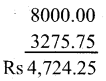The amount left Ramegowda Rs 4,724.25

Question 3.
Kishan had Rs.10,000 in his bank account. He withdrew Rs.5,475.5 for his expenses. Later he deposited Rs. 3,280.75. Find the amount left in his account in the bank?
Krishna had an amount – Rs 10,000.00
Krishna deposit an amount – Rs 3,280.75
Total amount deposit in the bank – 13,280.75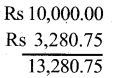Krishna withdraw an amount – Rs 5,475.50
The amount left with Krishna – Rs 7,805.25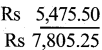Question 4.
Salma bought 20 chocolates at Rs.22 each. She gave the shopkeeper Rs. 500 note. What is the change she hds to get from the shopkeeper?
Sahan bought 20 chocolates
Each chocolate of cost Rs 22
The cost of 20 chocolate = 20 x 22 = Rs 440
Sahana gave Rs 500 to the shopkeeper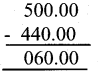Sahan Rs 60 from the shopkeeperQuestion 5.
Raghu had Rs.25,000 in his account in the bank. He later deposited Rs. 15,000 to his account. He took 8 gift cheques of equal value for the total amount from the bank. What is the amount of each gift cheque?
Raghu had an amount Rs 25,000
Raghu deposit Rs 15,000 to his account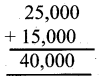Raghu took 8 gifts cheques of equal value for the total amount $$\frac{4000}{8}$$ = Rs 5000
The amount of each gift cheques Rs 5000

### KSEEB Class 5 Maths Money Ex 5.4

I. Check and correct the bills given below:

1.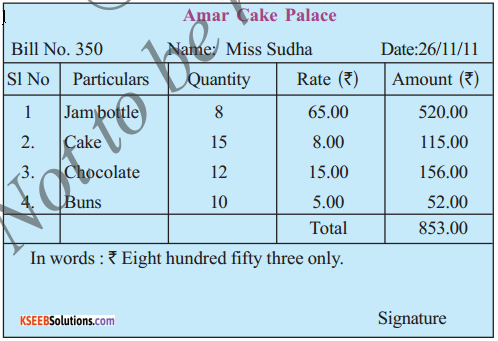2. Mrs. Uma bought the following articles from Sunny Enterprises.

• 5 instrument boxes at Rs. 38 each 12 pencils at Rs. 3 each
• 6 boxes of crayons at Rs.12 each.
• 8 paint boxes at Rs. 25 each.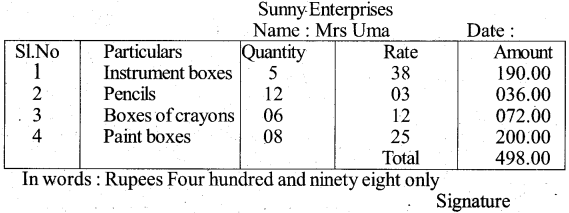3. Miss Rita bought the following items for the school Annual Day from megha Stationaries:

• 25 sheets of colour paper at Rs. 9 each
• 18 sheets of gold colour paper at Rs. 19 each
• 12 sheets of silver colour paper at Rs. 18 each.
• 2 tubes of glue stick at Rs. 30 each.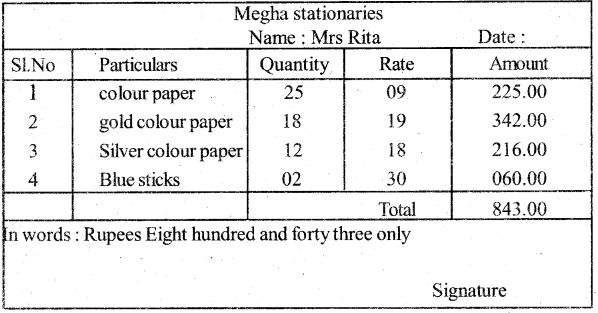4. Ranbir Singh bought the following articles from Hanuman Hardware shop.

• 2 hammers at Rs.85 each.
• 6 boxes of nails at Rs. 25 each.
• 8 boxes of screws at Rs.30 each box.
• 2 spanners at Rs.175 each.